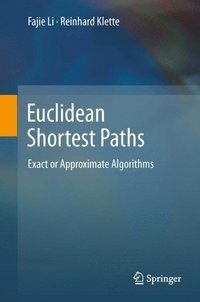Fler böcker inom
Format
E-bok
Filformat
PDF-böcker lämpar sig inte för läsning på små skärmar, t ex mobiler.
Språk
Engelska
Utgivningsdatum
2011-11-03
Förlag
Springer London
ISBN
9781447122562

### Du kanske gillar# Euclidean Shortest Paths (e-bok)

Exact or Approximate Algorithms

E-bok (PDF - DRM), Engelska, 2011-11-03
1549
Läs i vår app för iPhone, iPad och Android
Finns även som
• Skickas inom 10-15 vardagar
1569
• Skickas inom 10-15 vardagar
1719
The Euclidean shortest path (ESP) problem asks the question: what is the path of minimum length connecting two points in a 2- or 3-dimensional space? Variants of this industrially-significant computational geometry problem also require the path to pass through specified areas and avoid defined obstacles.This unique text/reference reviews algorithms for the exact or approximate solution of shortest-path problems, with a specific focus on a class of algorithms called rubberband algorithms. Discussing each concept and algorithm in depth, the book includes mathematical proofs for many of the given statements. Suitable for a second- or third-year university algorithms course, the text enables readers to understand not only the algorithms and their pseudocodes, but also the correctness proofs, the analysis of time complexities, and other related topics.Topics and features: provides theoretical and programming exercises at the end of each chapter; presents a thorough introduction to shortest paths in Euclidean geometry, and the class of algorithms called rubberband algorithms; discusses algorithms for calculating exact or approximate ESPs in the plane; examines the shortest paths on 3D surfaces, in simple polyhedrons and in cube-curves; describes the application of rubberband algorithms for solving art gallery problems, including the safari, zookeeper, watchman, and touring polygons route problems; includes lists of symbols and abbreviations, in addition to other appendices.This hands-on guide will be of interest to undergraduate students in computer science, IT, mathematics, and engineering. Programmers, mathematicians, and engineers dealing with shortest-path problems in practical applications will also find the book a useful resource.

## Kundrecensioner

Har du läst boken? Sätt ditt betyg »